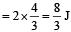Courses

# Test: Work, Energy And Power 2 - From Past 28 Years Questions

## 30 Questions MCQ Test Physics 28 Years Past year papers for NEET/AIPMT Class 11 | Test: Work, Energy And Power 2 - From Past 28 Years Questions

Description
This mock test of Test: Work, Energy And Power 2 - From Past 28 Years Questions for NEET helps you for every NEET entrance exam. This contains 30 Multiple Choice Questions for NEET Test: Work, Energy And Power 2 - From Past 28 Years Questions (mcq) to study with solutions a complete question bank. The solved questions answers in this Test: Work, Energy And Power 2 - From Past 28 Years Questions quiz give you a good mix of easy questions and tough questions. NEET students definitely take this Test: Work, Energy And Power 2 - From Past 28 Years Questions exercise for a better result in the exam. You can find other Test: Work, Energy And Power 2 - From Past 28 Years Questions extra questions, long questions & short questions for NEET on EduRev as well by searching above.
QUESTION: 1

### The co-efficient of restitution e for a perfectly elastic collision is 

Solution:

e = | v1 – v2 |/ | u1 – u2 | which is 1 for a perfectly elastic collision.

QUESTION: 2

### A bullet of mass  10g leaves a rifle at an initial velocity of 1000 m/s and strikes the earth at the same level with a velocity of 500 m/s. The work done in joules overcoming the resistance of air will be

Solution: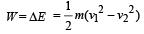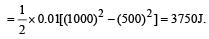QUESTION: 3

### How much water, a pump of 2 kW can raise in one minute to a height of 10 m, take g = 10 m/s2?      

Solution: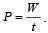Here, P = 2kW = 2000 W..
W = Mgh = M × 10 × 10 = 100 M and t = 60 s.
This gives, M = 1200 kg Its volume = 1200 litre as 1 litre of water contains 1 kg of its mass.

QUESTION: 4

Two identical balls A and B moving with velocities +0.5 m/s and –0.3 m/s respectively, collide head on elastically. The velocities of the balls A and B after collision, will be, respectively 

Solution:

When the identical balls collide head-on, their velocities are exchanged.

QUESTION: 5

A position dependent force, F = (7 – 2x + 3x2) N acts on a small body of mass 2 kg and displaces it from x = 0 to x = 5 m. Work done in joule is 

Solution: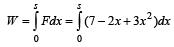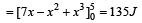QUESTION: 6

Two masses of 1g and 9g are moving with equal kinetic energies. The ratio of the magnitudes of their respective linear momenta is 

Solution: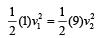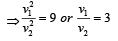Ratio of their linear momenta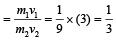QUESTION: 7

Consider a car moving along a straight horizantal road with a speed of 72 km/h. If the coefficient of static friction between road and tyres is 0.5, the shortest distance in which the car can be stopped is

Solution:

Force due to friction = kinetic energy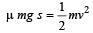[Here, v = 72 km/h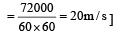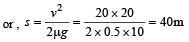QUESTION: 8

If the momentum of a body is increased by 50%, then the percentage increase in its kinetic energy is

Solution:

Initial momentum (p1) = p; Final momentum

(p2) = 1.5 p and initial kinetic energy (K1) = K.

Kinetic energy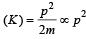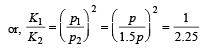or, K2 = 2.25 K.
Therefore, increase in kinetic energy is 2.25 K – K = 1.25 K or 125%.

QUESTION: 9

The kinetic energy acquired by a mass (m) in travelling distance (s) starting from rest under the action of a constant force is directly proportional to [1994, 1996]

Solution: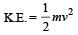Further, v2 = u2 + 2as = 0 + 2ad = 2ad = 2(F/m)d

Hence, K.E. =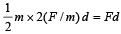or, K.E. acquired = Work done

= F × d = constant.i.e., it is independent of mass m.

QUESTION: 10

A body of mass m moving with velocity 3 km/h collides with a body of mass 2 m at rest. Now the coalesced mass starts to move with a velocity 

Solution:

Applying law of conservation of momentum,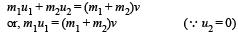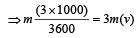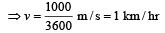QUESTION: 11

Two bodies of masses m and 4 m ar e moving with equal K.E. The ratio of their linear momenta is

Solution:

m1 = m, m2 = 4 m K . E1 = K . E2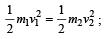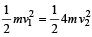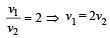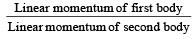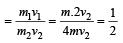QUESTION: 12

A molecule of mass m of an ideal gas collides with the wall of a vessel with a velocity v and returns back with the same velocity. The change in linear momentum of molecule is 

Solution: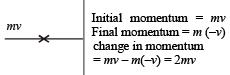QUESTION: 13

A metal ball of mass 2 kg moving with a velocity of 36 km/h has a head on collision with a stationary ball of mass 3 kg. If after the collision, the two balls move together, the loss in kinetic energy due to collision is 

Solution:

Applying conservation of momen tum,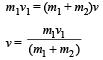Here, v1 = 36 km/hr = 10 m/s m1 = 2 kg, m2 = 3 kg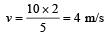K.E. (initial) =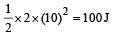K.E. (Final) =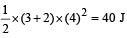Loss in K.E. = 100 – 40 = 60 J

QUESTION: 14

Two equal masses m1 and m2 moving along the same straight line with velocities + 3 m/s and – 5m/s respectively, collide elastically.Their velocities after the collision will be respectively. 

Solution:

In elastic collision, the velocities get in ter changed if the colliding objects have equal masses.

QUESTION: 15

A force acts on a 30 gm particle in such a way that the position of the particle as a function of time is given by x = 3t – 4t2 + t3, where x is in metres and t is in seconds. The work done during the first 4 seconds is 

Solution:

x = 3t –4t2 + t3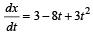Acceleration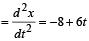Acceleration after 4sec = –8 + 6 × 4 = 16 ms–2
Displacement in 4sec = 3 ×4 – 4 × 42 + 43 = 12 m

∴  Work = Force × displacement = Mass × acc. × disp. = 3 × 10–3 × 16 × 12 = 576 mJ

QUESTION: 16

A rubber ball is dropped from a height of 5m on a plane, where the acceleration due to gravity is not shown. On bouncing it rises to 1.8 m. The ball loses its velocity on bouncing by a factor of 

Solution:

Accordin g to principle of conservation of energy Loss in potential energy = Gain in kinetic energy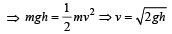If  h1 and h2 are initial and final heights, then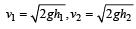Loss in velocity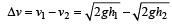∴  Fractional loss in velocity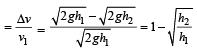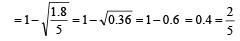QUESTION: 17

Two bodies with kinetic energies in the r atio 4 : 1 are moving with equal linear momentum. The ratio of their masses is 

Solution: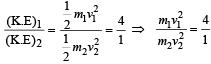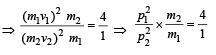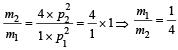[Given: p1=p2]

QUESTION: 18

A 3 kg ball strikes a heavy rigid wall with a speed of 10 m/s at an angle of 60º. It gets reflected with the same speed and angle as shown here. If the ball is in contact with the wall for 0.20s, what is the average force exerted on the ball by the wall?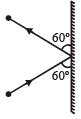Solution:

Change in momentum along the wall = mv cos60º – mv cos 60º = 0
Change in momentum perpendicular to the wall
= mv sin60º – (– mv sin60º) = 2mv sin60º

∴ Applied force =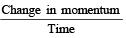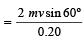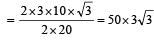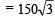newton

QUESTION: 19

A bomb of mass 1 kg is thr own vertically upwards with a speed of 100 m/s. After 5 seconds it explodes into two fragments. One fragment of mass 400 gm is found to go down with a speed of 25 m/s. What will happen to the second fragment just after the explosion? (g = 10 m/s2) 

Solution:

Speed of bomb after 5 second, v = u – gt  = 100 –10×5 = 50m/s

Momentum of 400 g fragment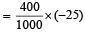[downward]

Momentum of 600g fragment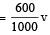Momentum of bomb = 1 × 50 = 50

From conservation of momentum Total momentum before splitting = total momentum after splitting.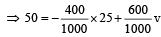⇒ v = 100 m/s [upward]

QUESTION: 20

In a simple pendulum of length l the bob is pulled aside from its equilibrium position through an angle θ and then released. The bob passes through the equilibrium position with speed.     

Solution:

If l is length of pendulum and θ be angular amplitude then height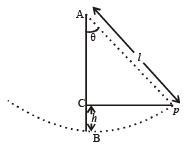h = AB AC = l – l cosθ = l(1 –  cosθ)
At extreme position, potential energy is maximum and kinetic energy is zero; At mean (equilibrium) position potential energy is zero and kinetic energy is maximum, so from principle of conservation of energy. (KE + PE ) at P = (KE + PE) at B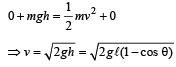QUESTION: 21

A force of 250 N is required to lift a 75 kg mass through a pulley system. In order to lift the mass through 3 m, the rope has to be pulled through 12m. Th e efficiency of system is 

Solution:

Efficiency =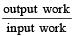i.e. Efficiency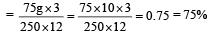QUESTION: 22

If the kinetic energy of a particle is increased by 300%, the momentum of the particle will increase by

Solution:

New K.E.,  E' = 4E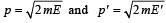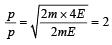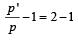[on substrating 1 in both sides.]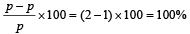QUESTION: 23

When a long sprin g is stretched by 2 cm, its potential energy is U. If the spring is stretched by 10 cm, the potential energy stored in it will be

Solution:

If k be the spring constant, then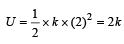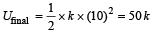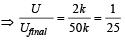⇒ U final = 25U

QUESTION: 24

A stationary particle explodes into two particles of masses m1 and m2 which move in opposite directions with velocities v1 and v2. The ratio of their kinetic energies E1/E2 is 

Solution:

From conservation law of momentum, before collision and after collision linear momentum (p) will be same. That is, initial momentum = final momentum.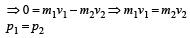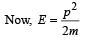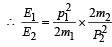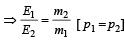QUESTION: 25

A mass of 0.5 kg moving with a speed of 1.5 m/ s on a horizontal smooth surface, collides with a nearly weightless spring of force constant k = 50 N/m. The  maximum compression of the spring would be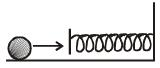Solution: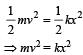or 0.5 × (1.5)2 = 50×x2

∴  x = 0.15 m

QUESTION: 26

A particle of mass m1 is moving with a velocity v1 and another particle of mass m2 is moving with a velocity v2. Both  of them have the same momentum but their different kinetic energies are E1 and E2 respectively. If m1 > m2 then 

Solution: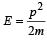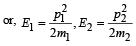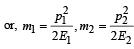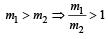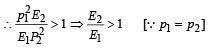or, E2 > E1

QUESTION: 27

A ball of mass 2 kg and another of mass 4 kg are dropped together from a 60 feet tall building.After a fall of 30 feet each towards earth, their respective kinetic energies will be in the ratio of 

Solution:

Since height is same for both balls, their velocities on reaching the ground will be same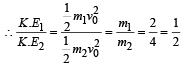QUESTION: 28

A force F acting on an object varies with distance x as shown here. The force is in N and x in m. The work done by the force in moving the object fr om x = 0 to x = 6 m is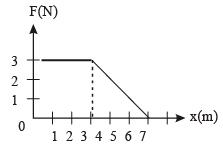Solution: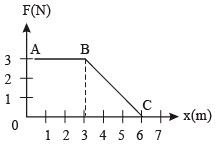Work done  = area under F-x graph = area of trapezium OABC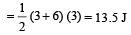QUESTION: 29

A bomb of mass 30 kg at rest explodes into two pieces of masses 18 kg and 12 kg. The velocity of 18 kg mass is 6 ms–1. The kinetic energy of the other mass is 

Solution:

From conservation of linear momentum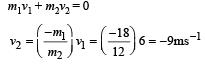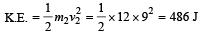QUESTION: 30

A body of  mass 3 kg is under a constant force which causes a displacements in metres in it, given by the relation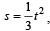, where t is in seconds.
Work done by the force in 2 seconds is 

Solution:

Acceleration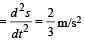Force acting on the body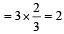newton

Displacement in 2 secs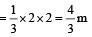Work done